# RD Sharma Solutions for Class 8 Maths Chapter 1 - Rational Numbers Exercise 1.2

RD Sharma Solutions for Class 8 Maths Exercise 1.2 Chapter 1, Rational Numbers. Students can download from the links provided below. This set of solutions are designed by our expert team in order to help students excel in their exam with flying colours. In Exercise 1.2 of RD Sharma Class 8 Maths Solution, we shall discuss problems based on the properties of addition of rational numbers which includes closure property, commutativity, associativity, the existence of additive identity.

## Download PDF of RD Sharma Solutions for Class 8 Maths Exercise 1.2 Chapter 1 Rational Numbers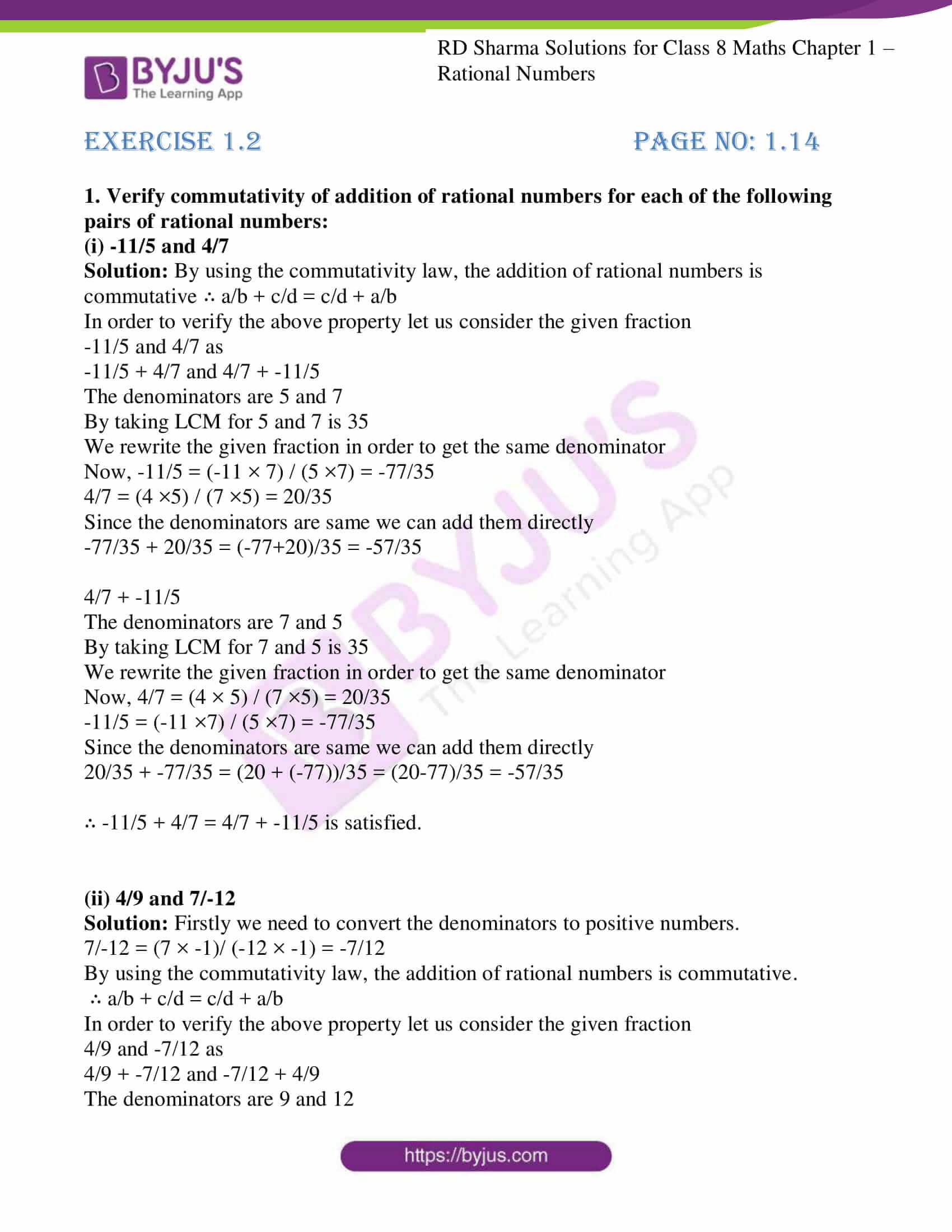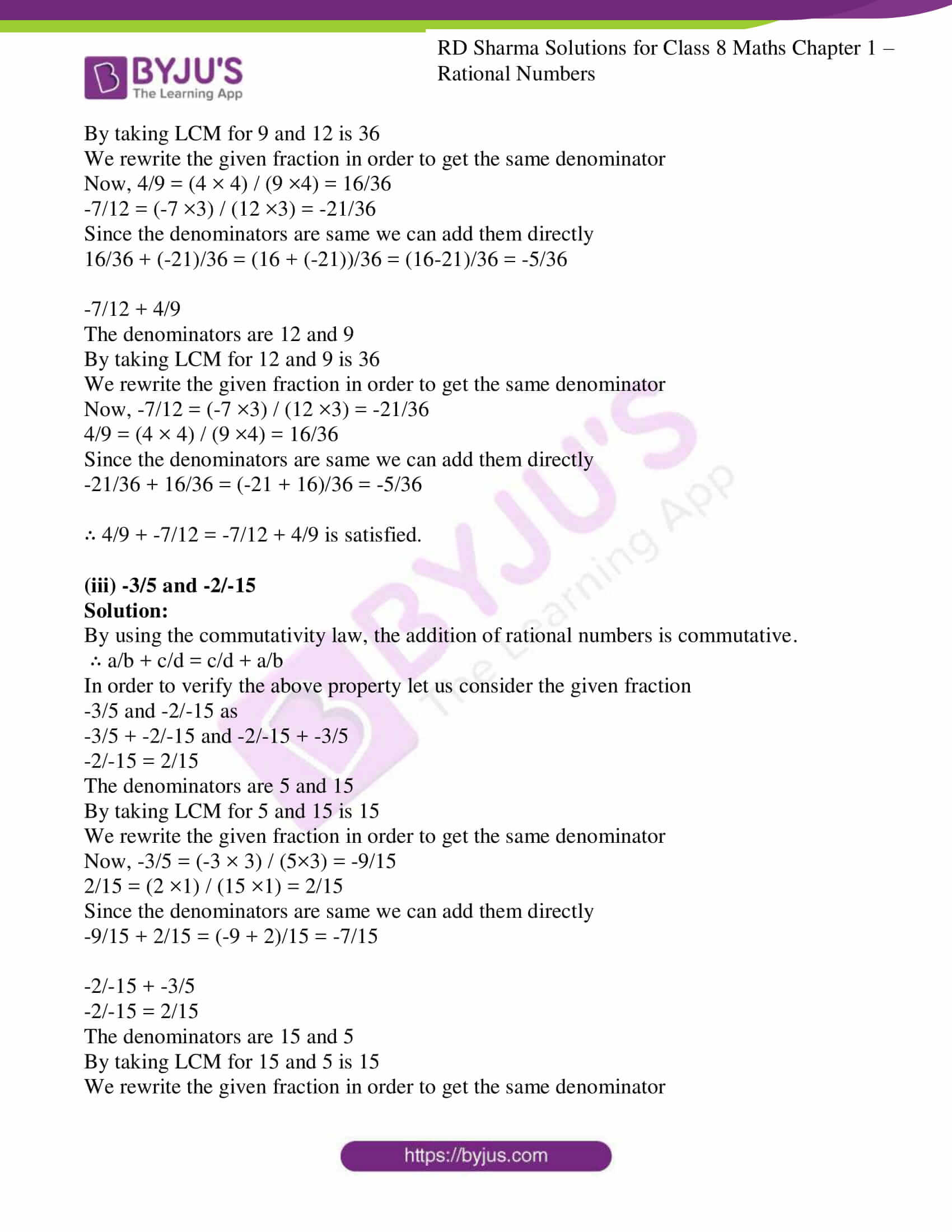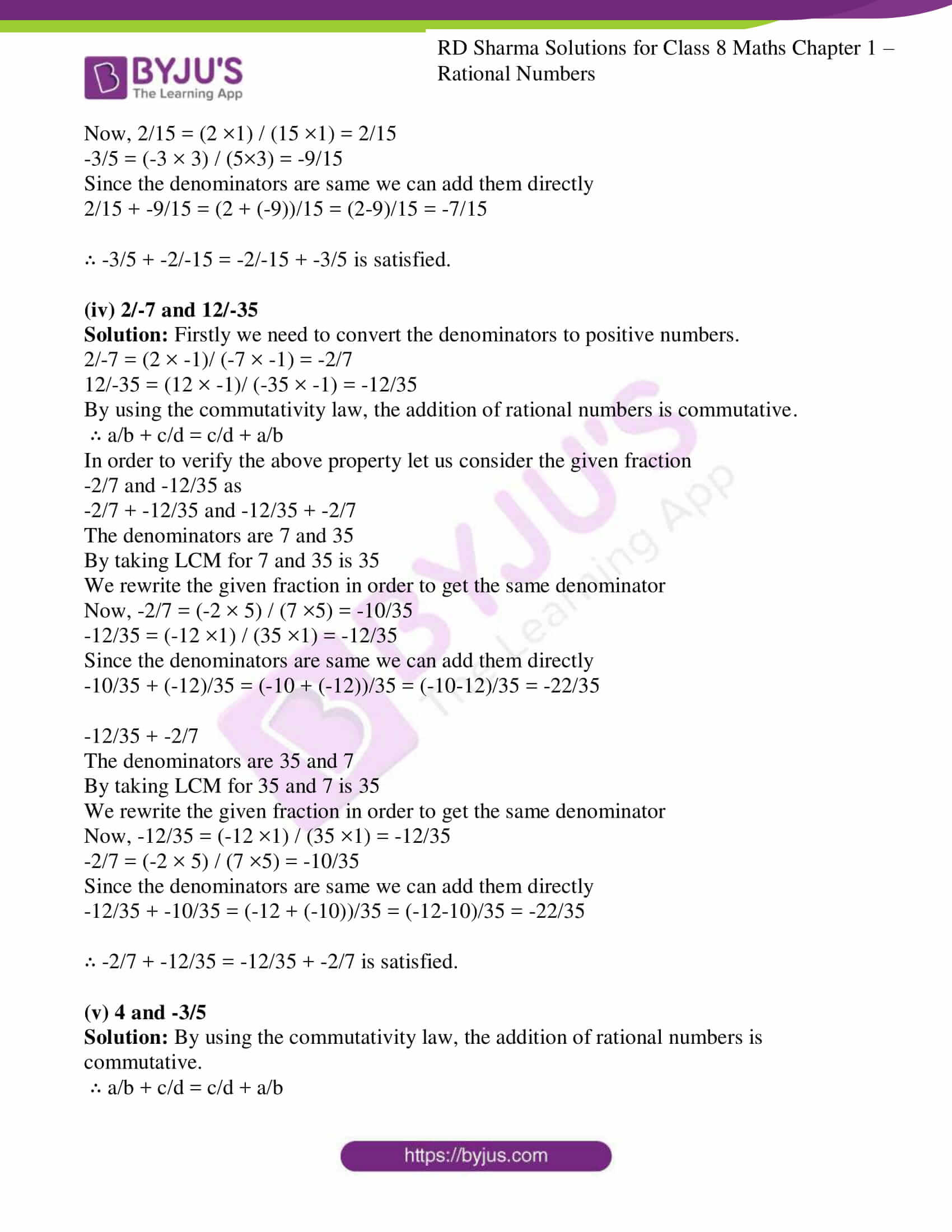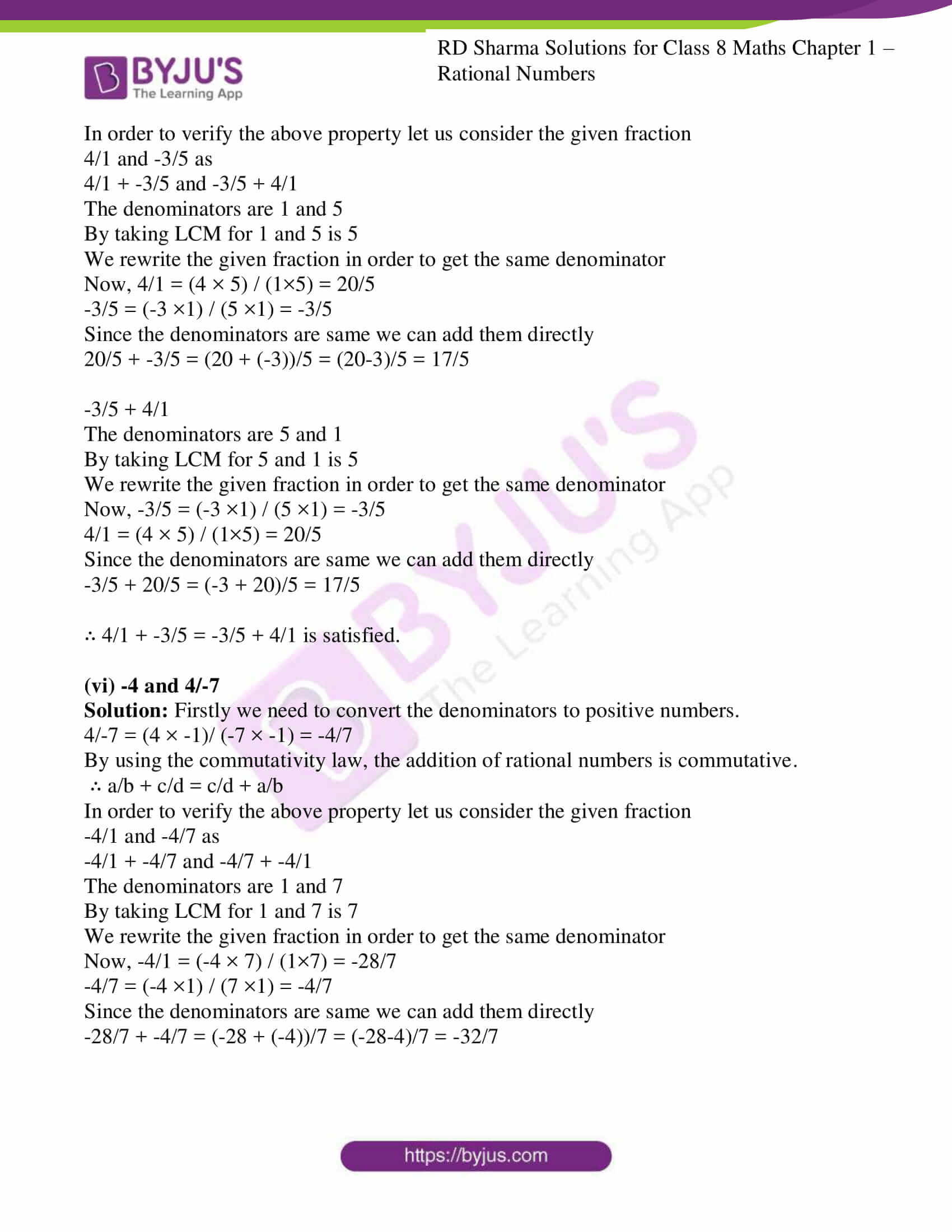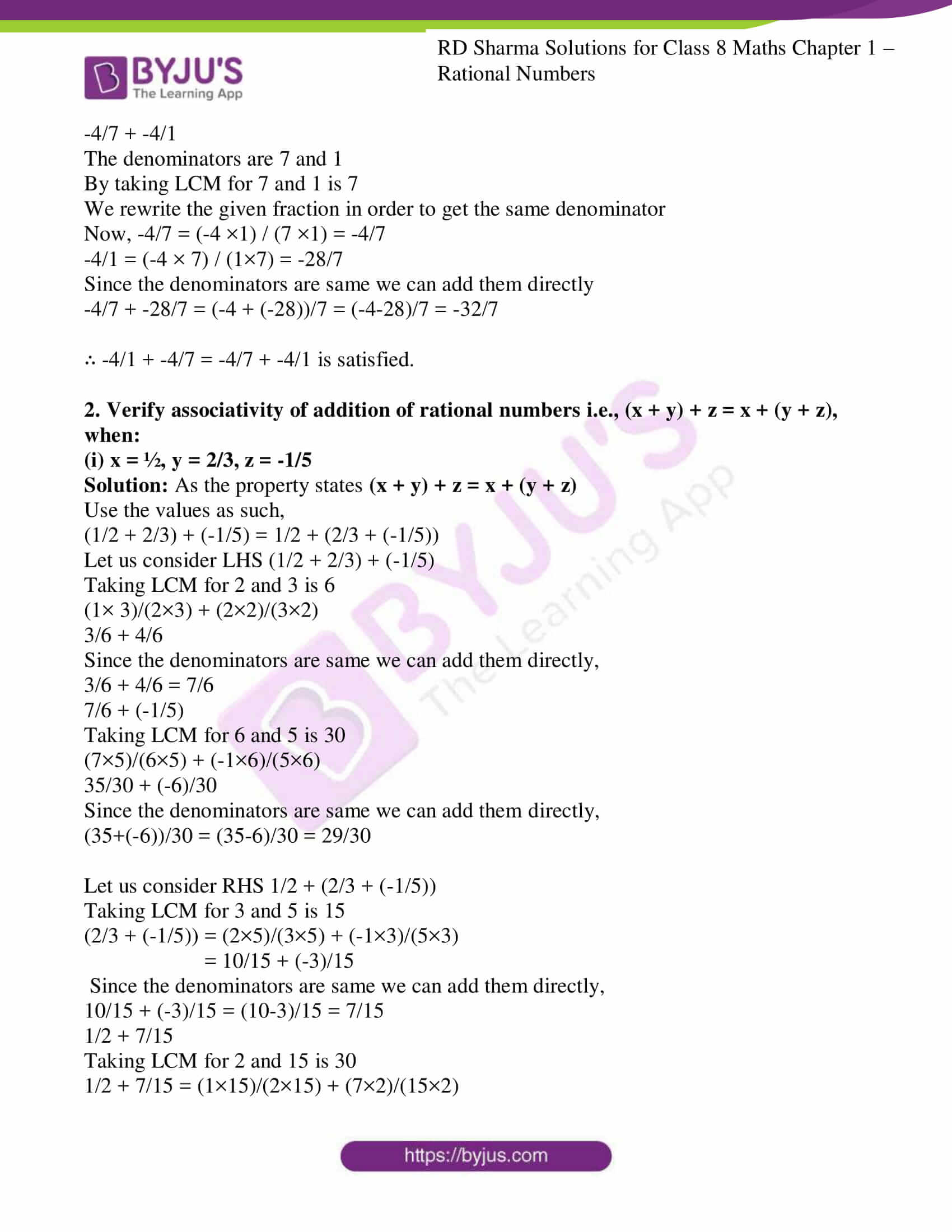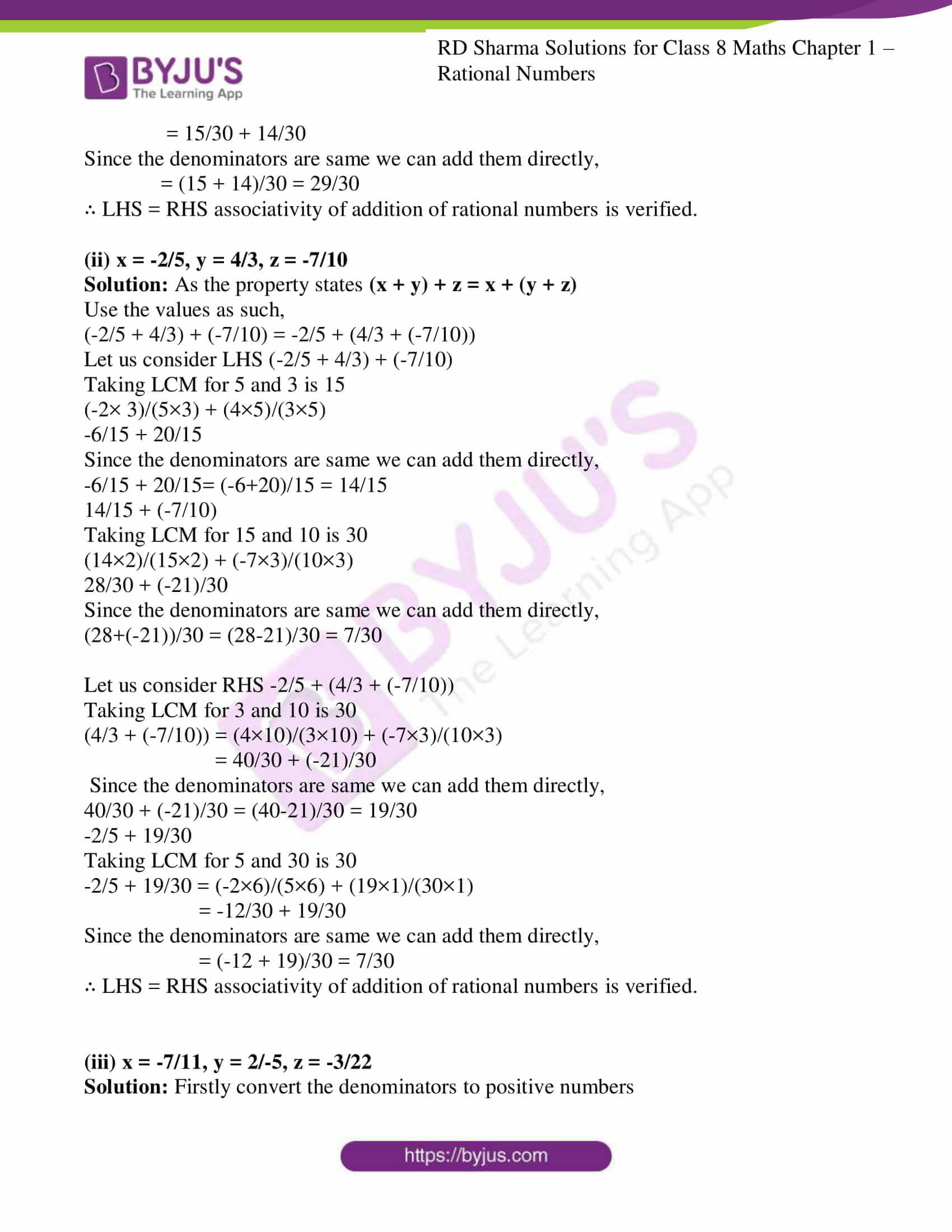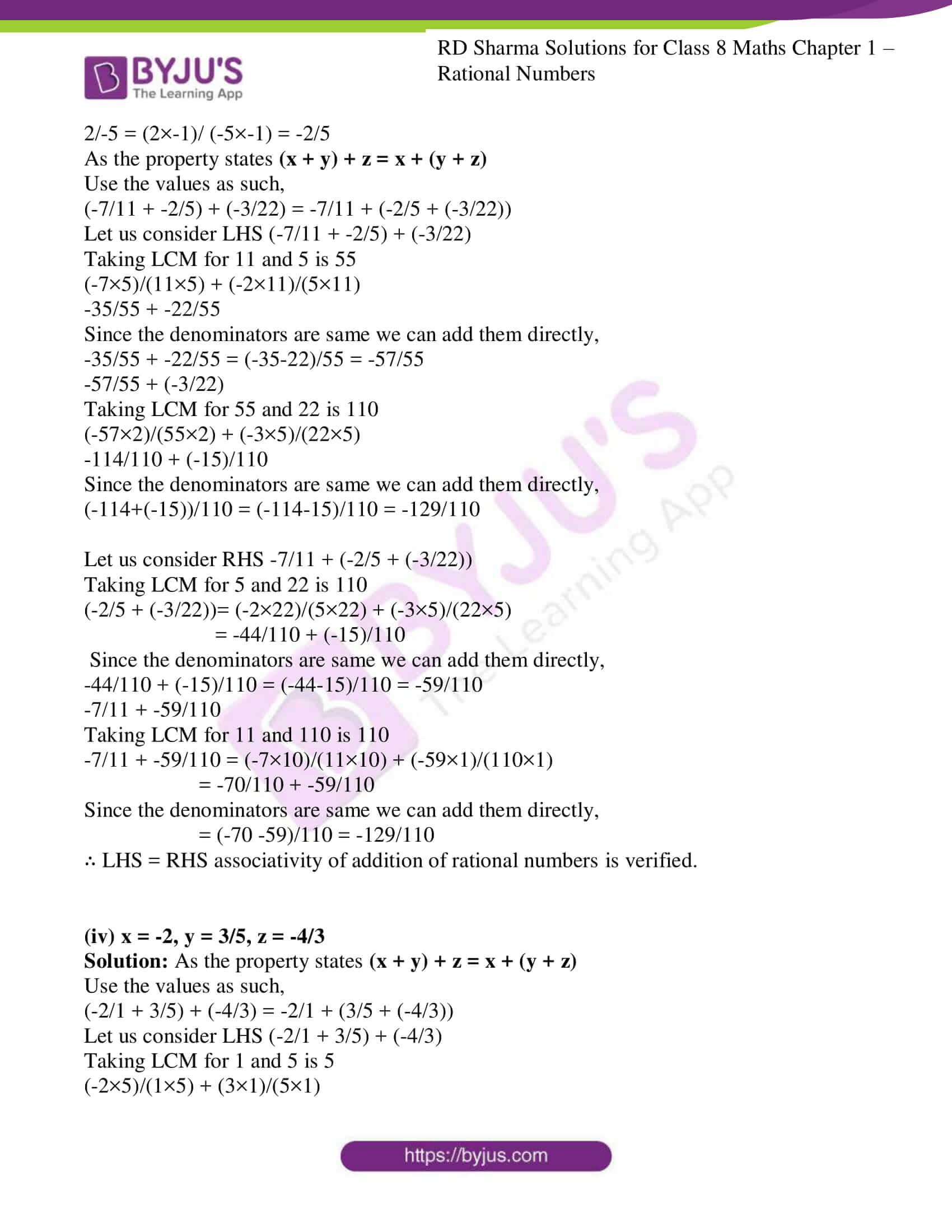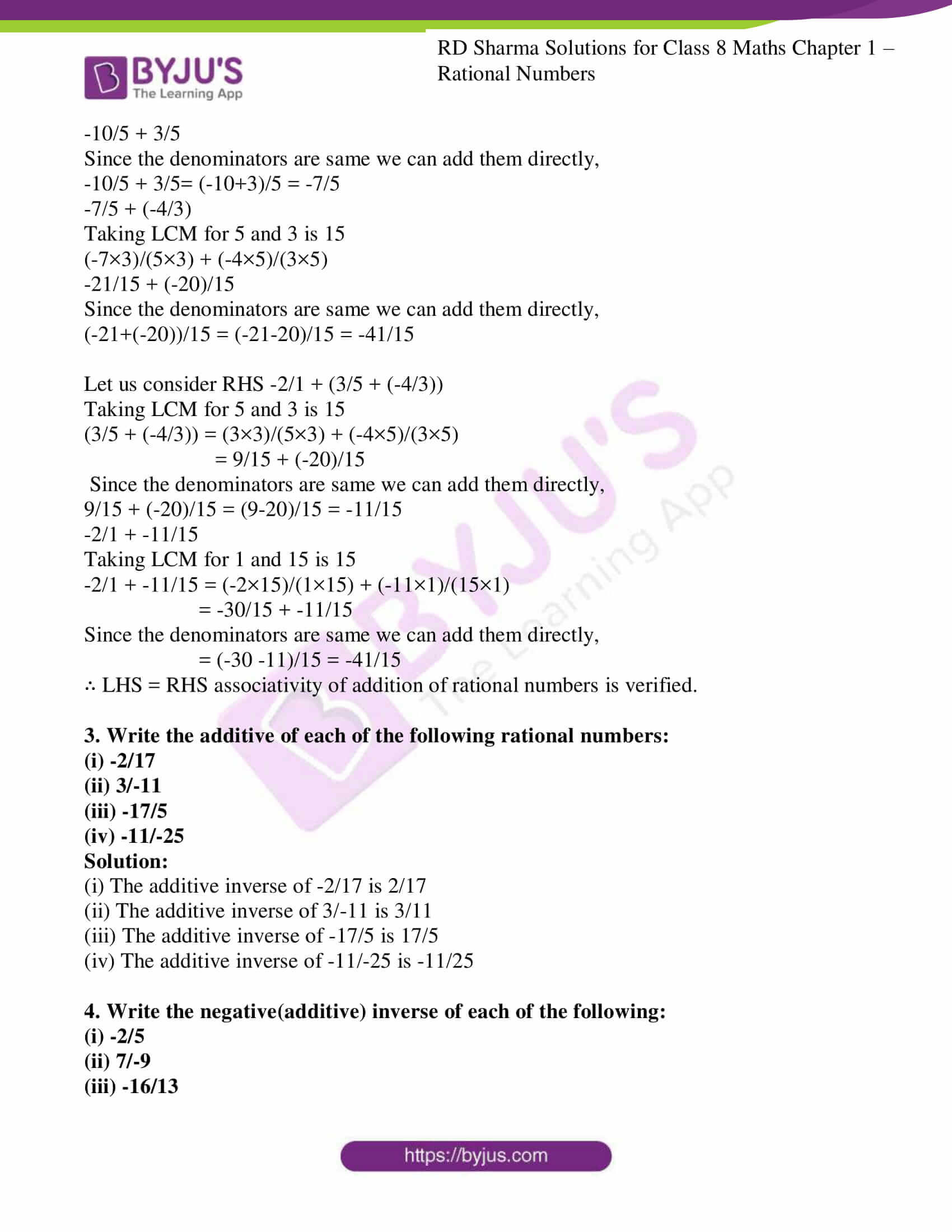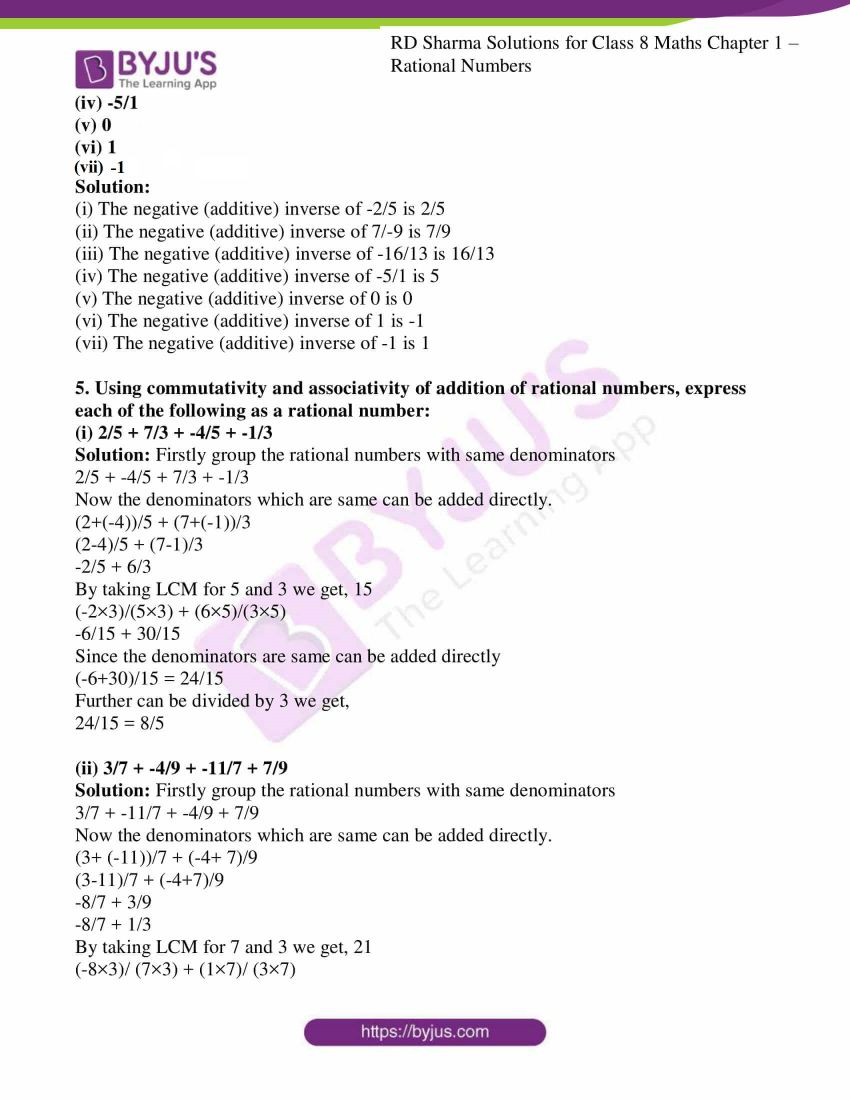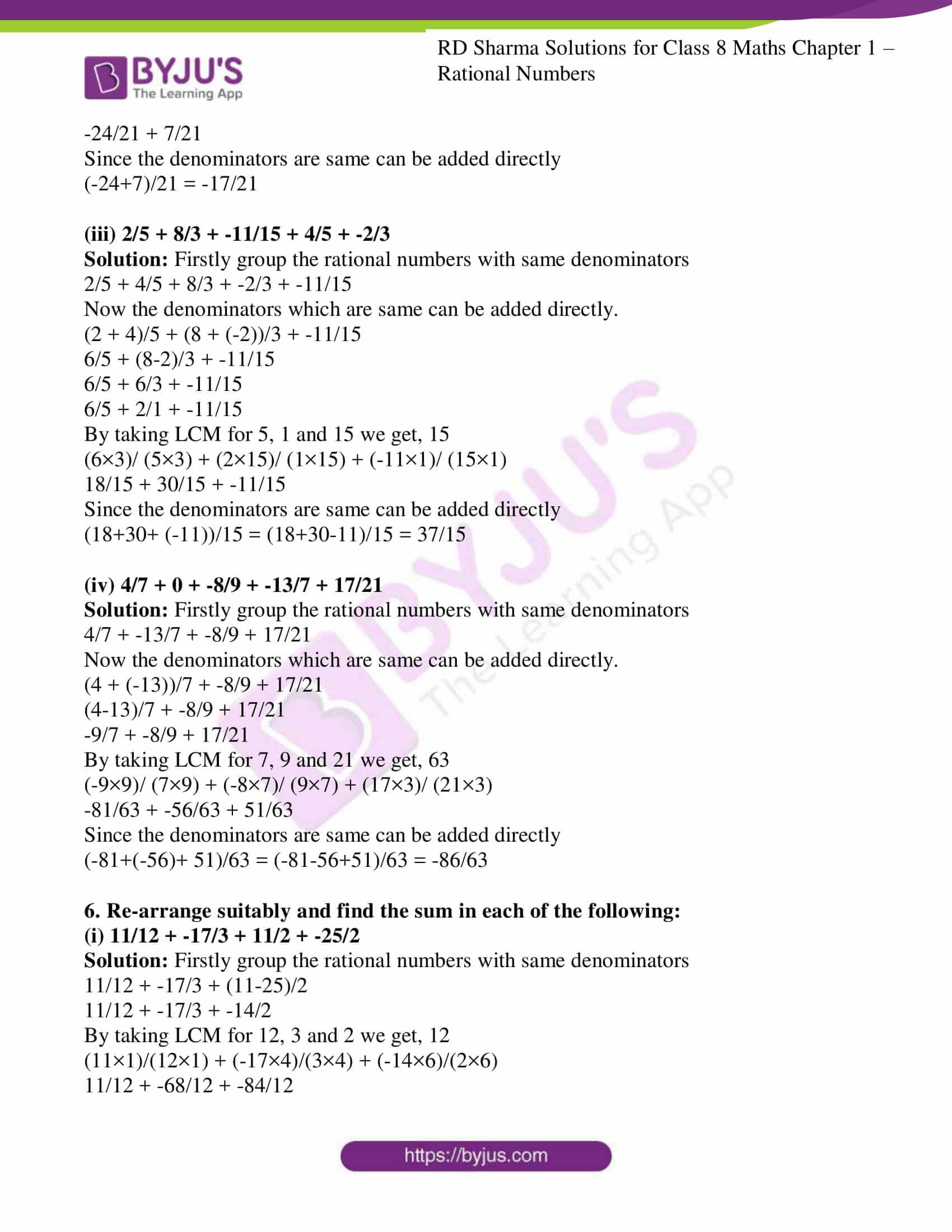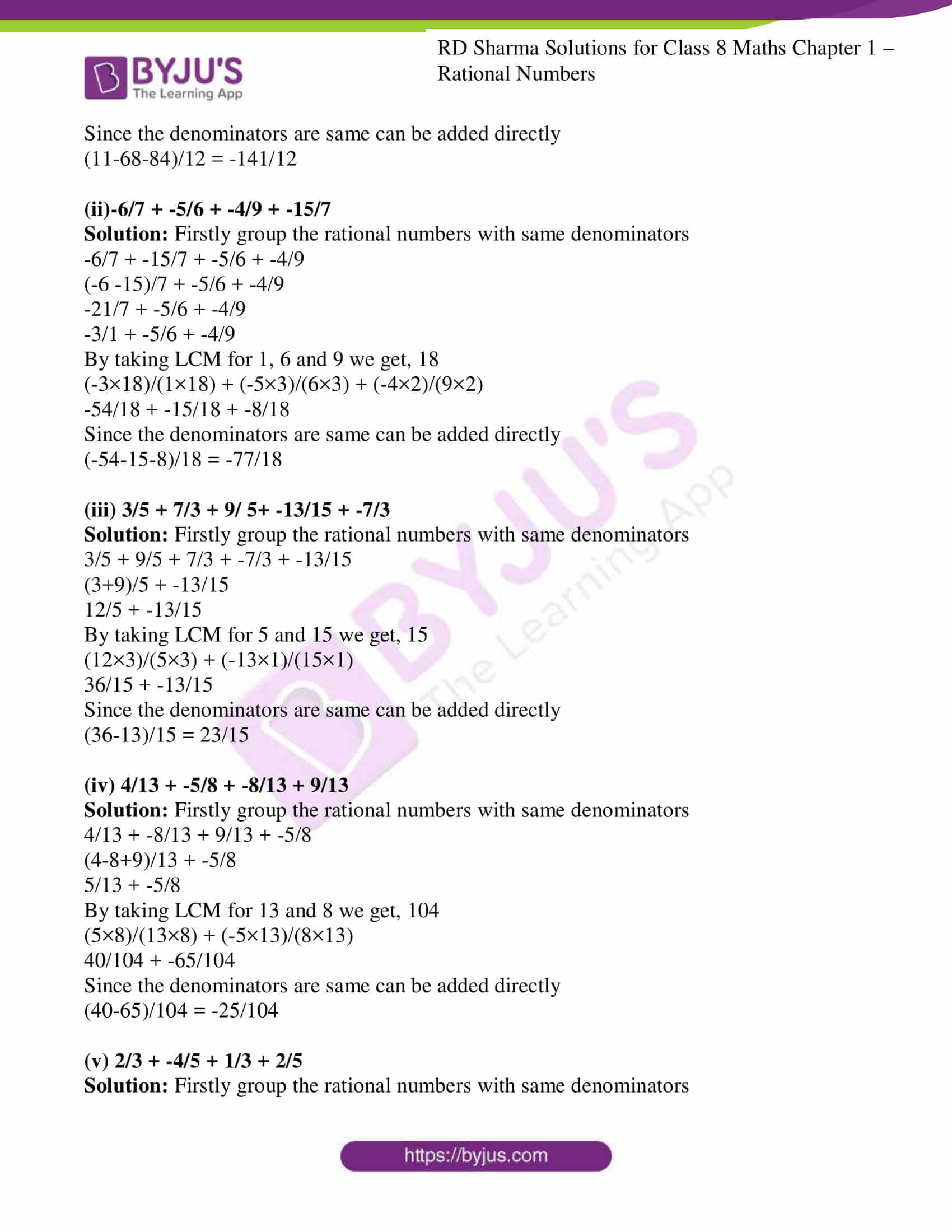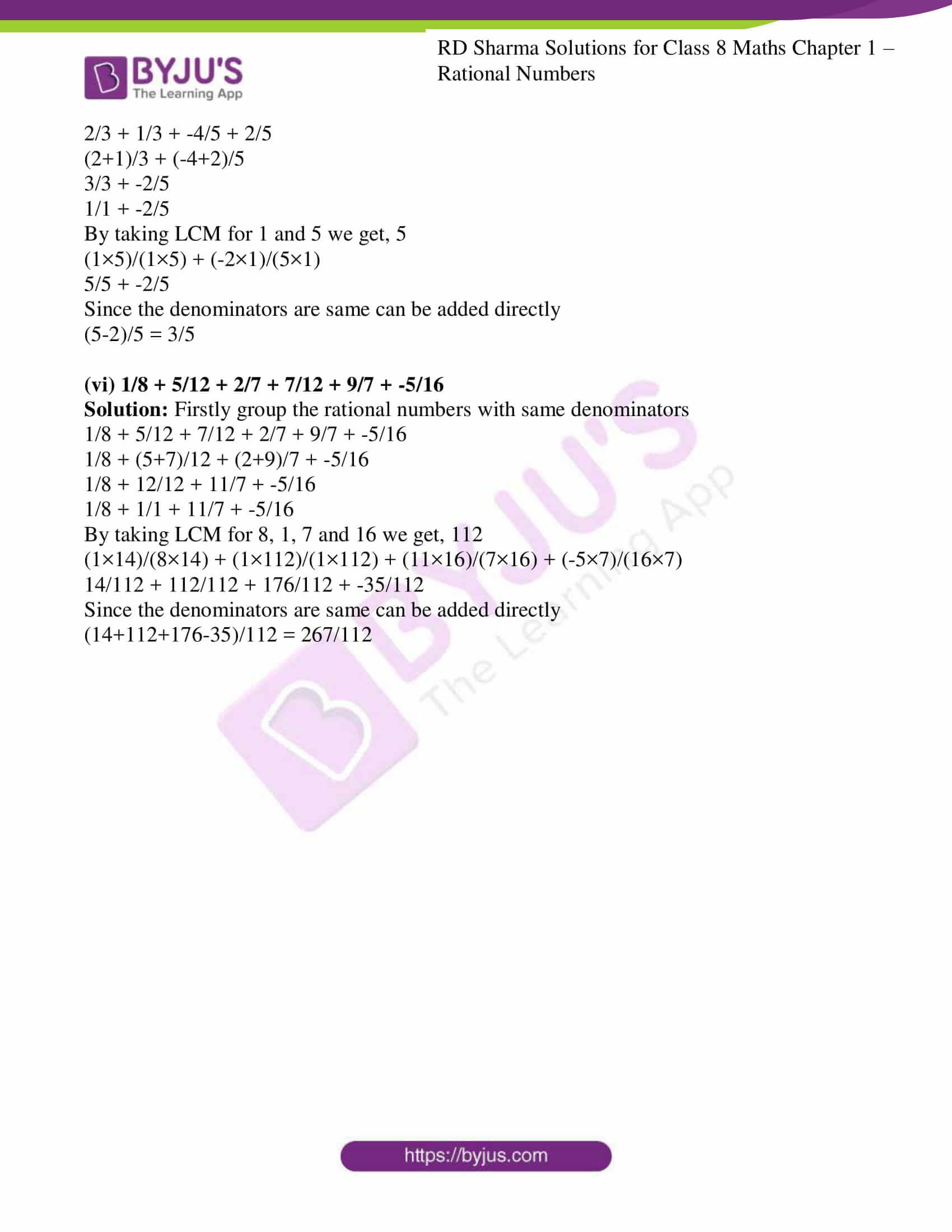### Access Answers to RD Sharma Solutions for Class 8 Maths Exercise 1.2 Chapter 1 Rational Numbers

1. Verify commutativity of addition of rational numbers for each of the following pairs of rational numbers:

(i) -11/5 and 4/7

Solution: By using the commutativity law, the addition of rational numbers is commutative ∴ a/b + c/d = c/d + a/b

In order to verify the above property let us consider the given fraction

-11/5 and 4/7 as

-11/5 + 4/7 and 4/7 + -11/5

The denominators are 5 and 7

By taking LCM for 5 and 7 is 35

We rewrite the given fraction in order to get the same denominator

Now, -11/5 = (-11 × 7) / (5 ×7) = -77/35

4/7 = (4 ×5) / (7 ×5) = 20/35

Since the denominators are same we can add them directly

-77/35 + 20/35 = (-77+20)/35 = -57/35

4/7 + -11/5

The denominators are 7 and 5

By taking LCM for 7 and 5 is 35

We rewrite the given fraction in order to get the same denominator

Now, 4/7 = (4 × 5) / (7 ×5) = 20/35

-11/5 = (-11 ×7) / (5 ×7) = -77/35

Since the denominators are same we can add them directly

20/35 + -77/35 = (20 + (-77))/35 = (20-77)/35 = -57/35

∴ -11/5 + 4/7 = 4/7 + -11/5 is satisfied.

(ii) 4/9 and 7/-12

Solution: Firstly we need to convert the denominators to positive numbers.

7/-12 = (7 × -1)/ (-12 × -1) = -7/12

By using the commutativity law, the addition of rational numbers is commutative.

∴ a/b + c/d = c/d + a/b

In order to verify the above property let us consider the given fraction

4/9 and -7/12 as

4/9 + -7/12 and -7/12 + 4/9

The denominators are 9 and 12

By taking LCM for 9 and 12 is 36

We rewrite the given fraction in order to get the same denominator

Now, 4/9 = (4 × 4) / (9 ×4) = 16/36

-7/12 = (-7 ×3) / (12 ×3) = -21/36

Since the denominators are same we can add them directly

16/36 + (-21)/36 = (16 + (-21))/36 = (16-21)/36 = -5/36

-7/12 + 4/9

The denominators are 12 and 9

By taking LCM for 12 and 9 is 36

We rewrite the given fraction in order to get the same denominator

Now, -7/12 = (-7 ×3) / (12 ×3) = -21/36

4/9 = (4 × 4) / (9 ×4) = 16/36

Since the denominators are same we can add them directly

-21/36 + 16/36 = (-21 + 16)/36 = -5/36

∴ 4/9 + -7/12 = -7/12 + 4/9 is satisfied.

(iii) -3/5 and -2/-15

Solution:

By using the commutativity law, the addition of rational numbers is commutative.

∴ a/b + c/d = c/d + a/b

In order to verify the above property let us consider the given fraction

-3/5 and -2/-15 as

-3/5 + -2/-15 and -2/-15 + -3/5

-2/-15 = 2/15

The denominators are 5 and 15

By taking LCM for 5 and 15 is 15

We rewrite the given fraction in order to get the same denominator

Now, -3/5 = (-3 × 3) / (5×3) = -9/15

2/15 = (2 ×1) / (15 ×1) = 2/15

Since the denominators are same we can add them directly

-9/15 + 2/15 = (-9 + 2)/15 = -7/15

-2/-15 + -3/5

-2/-15 = 2/15

The denominators are 15 and 5

By taking LCM for 15 and 5 is 15

We rewrite the given fraction in order to get the same denominator

Now, 2/15 = (2 ×1) / (15 ×1) = 2/15

-3/5 = (-3 × 3) / (5×3) = -9/15

Since the denominators are same we can add them directly

2/15 + -9/15 = (2 + (-9))/15 = (2-9)/15 = -7/15

∴ -3/5 + -2/-15 = -2/-15 + -3/5 is satisfied.

(iv) 2/-7 and 12/-35

Solution: Firstly we need to convert the denominators to positive numbers.

2/-7 = (2 × -1)/ (-7 × -1) = -2/7

12/-35 = (12 × -1)/ (-35 × -1) = -12/35

By using the commutativity law, the addition of rational numbers is commutative.

∴ a/b + c/d = c/d + a/b

In order to verify the above property let us consider the given fraction

-2/7 and -12/35 as

-2/7 + -12/35 and -12/35 + -2/7

The denominators are 7 and 35

By taking LCM for 7 and 35 is 35

We rewrite the given fraction in order to get the same denominator

Now, -2/7 = (-2 × 5) / (7 ×5) = -10/35

-12/35 = (-12 ×1) / (35 ×1) = -12/35

Since the denominators are same we can add them directly

-10/35 + (-12)/35 = (-10 + (-12))/35 = (-10-12)/35 = -22/35

-12/35 + -2/7

The denominators are 35 and 7

By taking LCM for 35 and 7 is 35

We rewrite the given fraction in order to get the same denominator

Now, -12/35 = (-12 ×1) / (35 ×1) = -12/35

-2/7 = (-2 × 5) / (7 ×5) = -10/35

Since the denominators are same we can add them directly

-12/35 + -10/35 = (-12 + (-10))/35 = (-12-10)/35 = -22/35

∴ -2/7 + -12/35 = -12/35 + -2/7 is satisfied.

(v) 4 and -3/5

Solution: By using the commutativity law, the addition of rational numbers is commutative.

∴ a/b + c/d = c/d + a/b

In order to verify the above property let us consider the given fraction

4/1 and -3/5 as

4/1 + -3/5 and -3/5 + 4/1

The denominators are 1 and 5

By taking LCM for 1 and 5 is 5

We rewrite the given fraction in order to get the same denominator

Now, 4/1 = (4 × 5) / (1×5) = 20/5

-3/5 = (-3 ×1) / (5 ×1) = -3/5

Since the denominators are same we can add them directly

20/5 + -3/5 = (20 + (-3))/5 = (20-3)/5 = 17/5

-3/5 + 4/1

The denominators are 5 and 1

By taking LCM for 5 and 1 is 5

We rewrite the given fraction in order to get the same denominator

Now, -3/5 = (-3 ×1) / (5 ×1) = -3/5

4/1 = (4 × 5) / (1×5) = 20/5

Since the denominators are same we can add them directly

-3/5 + 20/5 = (-3 + 20)/5 = 17/5

∴ 4/1 + -3/5 = -3/5 + 4/1 is satisfied.

(vi) -4 and 4/-7

Solution: Firstly we need to convert the denominators to positive numbers.

4/-7 = (4 × -1)/ (-7 × -1) = -4/7

By using the commutativity law, the addition of rational numbers is commutative.

∴ a/b + c/d = c/d + a/b

In order to verify the above property let us consider the given fraction

-4/1 and -4/7 as

-4/1 + -4/7 and -4/7 + -4/1

The denominators are 1 and 7

By taking LCM for 1 and 7 is 7

We rewrite the given fraction in order to get the same denominator

Now, -4/1 = (-4 × 7) / (1×7) = -28/7

-4/7 = (-4 ×1) / (7 ×1) = -4/7

Since the denominators are same we can add them directly

-28/7 + -4/7 = (-28 + (-4))/7 = (-28-4)/7 = -32/7

-4/7 + -4/1

The denominators are 7 and 1

By taking LCM for 7 and 1 is 7

We rewrite the given fraction in order to get the same denominator

Now, -4/7 = (-4 ×1) / (7 ×1) = -4/7

-4/1 = (-4 × 7) / (1×7) = -28/7

Since the denominators are same we can add them directly

-4/7 + -28/7 = (-4 + (-28))/7 = (-4-28)/7 = -32/7

∴ -4/1 + -4/7 = -4/7 + -4/1 is satisfied.

2. Verify associativity of addition of rational numbers i.e., (x + y) + z = x + (y + z), when:

(i) x = ½, y = 2/3, z = -1/5

Solution: As the property states (x + y) + z = x + (y + z)

Use the values as such,

(1/2 + 2/3) + (-1/5) = 1/2 + (2/3 + (-1/5))

Let us consider LHS (1/2 + 2/3) + (-1/5)

Taking LCM for 2 and 3 is 6

(1× 3)/(2×3) + (2×2)/(3×2)

3/6 + 4/6

Since the denominators are same we can add them directly,

3/6 + 4/6 = 7/6

7/6 + (-1/5)

Taking LCM for 6 and 5 is 30

(7×5)/(6×5) + (-1×6)/(5×6)

35/30 + (-6)/30

Since the denominators are same we can add them directly,

(35+(-6))/30 = (35-6)/30 = 29/30

Let us consider RHS 1/2 + (2/3 + (-1/5))

Taking LCM for 3 and 5 is 15

(2/3 + (-1/5)) = (2×5)/(3×5) + (-1×3)/(5×3)

= 10/15 + (-3)/15

Since the denominators are same we can add them directly,

10/15 + (-3)/15 = (10-3)/15 = 7/15

1/2 + 7/15

Taking LCM for 2 and 15 is 30

1/2 + 7/15 = (1×15)/(2×15) + (7×2)/(15×2)

= 15/30 + 14/30

Since the denominators are same we can add them directly,

= (15 + 14)/30 = 29/30

∴ LHS = RHS associativity of addition of rational numbers is verified.

(ii) x = -2/5, y = 4/3, z = -7/10

Solution: As the property states (x + y) + z = x + (y + z)

Use the values as such,

(-2/5 + 4/3) + (-7/10) = -2/5 + (4/3 + (-7/10))

Let us consider LHS (-2/5 + 4/3) + (-7/10)

Taking LCM for 5 and 3 is 15

(-2× 3)/(5×3) + (4×5)/(3×5)

-6/15 + 20/15

Since the denominators are same we can add them directly,

-6/15 + 20/15= (-6+20)/15 = 14/15

14/15 + (-7/10)

Taking LCM for 15 and 10 is 30

(14×2)/(15×2) + (-7×3)/(10×3)

28/30 + (-21)/30

Since the denominators are same we can add them directly,

(28+(-21))/30 = (28-21)/30 = 7/30

Let us consider RHS -2/5 + (4/3 + (-7/10))

Taking LCM for 3 and 10 is 30

(4/3 + (-7/10)) = (4×10)/(3×10) + (-7×3)/(10×3)

= 40/30 + (-21)/30

Since the denominators are same we can add them directly,

40/30 + (-21)/30 = (40-21)/30 = 19/30

-2/5 + 19/30

Taking LCM for 5 and 30 is 30

-2/5 + 19/30 = (-2×6)/(5×6) + (19×1)/(30×1)

= -12/30 + 19/30

Since the denominators are same we can add them directly,

= (-12 + 19)/30 = 7/30

∴ LHS = RHS associativity of addition of rational numbers is verified.

(iii) x = -7/11, y = 2/-5, z = -3/22

Solution: Firstly convert the denominators to positive numbers

2/-5 = (2×-1)/ (-5×-1) = -2/5

As the property states (x + y) + z = x + (y + z)

Use the values as such,

(-7/11 + -2/5) + (-3/22) = -7/11 + (-2/5 + (-3/22))

Let us consider LHS (-7/11 + -2/5) + (-3/22)

Taking LCM for 11 and 5 is 55

(-7×5)/(11×5) + (-2×11)/(5×11)

-35/55 + -22/55

Since the denominators are same we can add them directly,

-35/55 + -22/55 = (-35-22)/55 = -57/55

-57/55 + (-3/22)

Taking LCM for 55 and 22 is 110

(-57×2)/(55×2) + (-3×5)/(22×5)

-114/110 + (-15)/110

Since the denominators are same we can add them directly,

(-114+(-15))/110 = (-114-15)/110 = -129/110

Let us consider RHS -7/11 + (-2/5 + (-3/22))

Taking LCM for 5 and 22 is 110

(-2/5 + (-3/22))= (-2×22)/(5×22) + (-3×5)/(22×5)

= -44/110 + (-15)/110

Since the denominators are same we can add them directly,

-44/110 + (-15)/110 = (-44-15)/110 = -59/110

-7/11 + -59/110

Taking LCM for 11 and 110 is 110

-7/11 + -59/110 = (-7×10)/(11×10) + (-59×1)/(110×1)

= -70/110 + -59/110

Since the denominators are same we can add them directly,

= (-70 -59)/110 = -129/110

∴ LHS = RHS associativity of addition of rational numbers is verified.

(iv) x = -2, y = 3/5, z = -4/3

Solution: As the property states (x + y) + z = x + (y + z)

Use the values as such,

(-2/1 + 3/5) + (-4/3) = -2/1 + (3/5 + (-4/3))

Let us consider LHS (-2/1 + 3/5) + (-4/3)

Taking LCM for 1 and 5 is 5

(-2×5)/(1×5) + (3×1)/(5×1)

-10/5 + 3/5

Since the denominators are same we can add them directly,

-10/5 + 3/5= (-10+3)/5 = -7/5

-7/5 + (-4/3)

Taking LCM for 5 and 3 is 15

(-7×3)/(5×3) + (-4×5)/(3×5)

-21/15 + (-20)/15

Since the denominators are same we can add them directly,

(-21+(-20))/15 = (-21-20)/15 = -41/15

Let us consider RHS -2/1 + (3/5 + (-4/3))

Taking LCM for 5 and 3 is 15

(3/5 + (-4/3)) = (3×3)/(5×3) + (-4×5)/(3×5)

= 9/15 + (-20)/15

Since the denominators are same we can add them directly,

9/15 + (-20)/15 = (9-20)/15 = -11/15

-2/1 + -11/15

Taking LCM for 1 and 15 is 15

-2/1 + -11/15 = (-2×15)/(1×15) + (-11×1)/(15×1)

= -30/15 + -11/15

Since the denominators are same we can add them directly,

= (-30 -11)/15 = -41/15

∴ LHS = RHS associativity of addition of rational numbers is verified.

3. Write the additive of each of the following rational numbers:

(i) -2/17

(ii) 3/-11

(iii) -17/5

(iv) -11/-25

Solution:

(i) The additive inverse of -2/17 is 2/17

(ii) The additive inverse of 3/-11 is 3/11

(iii) The additive inverse of -17/5 is 17/5

(iv) The additive inverse of -11/-25 is -11/25

4. Write the negative(additive) inverse of each of the following:

(i) -2/5

(ii) 7/-9

(iii) -16/13

(iv) -5/1

(v) 0

(vi) 1

(vii) -1

Solution:

(i) The negative (additive) inverse of -2/5 is 2/5

(ii) The negative (additive) inverse of 7/-9 is 7/9

(iii) The negative (additive) inverse of -16/13 is 16/13

(iv) The negative (additive) inverse of -5/1 is 5

(v) The negative (additive) inverse of 0 is 0

(vi) The negative (additive) inverse of 1 is -1

(vii) The negative (additive) inverse of -1 is 1

5. Using commutativity and associativity of addition of rational numbers, express each of the following as a rational number:

(i) 2/5 + 7/3 + -4/5 + -1/3

Solution: Firstly group the rational numbers with same denominators

2/5 + -4/5 + 7/3 + -1/3

Now the denominators which are same can be added directly.

(2+(-4))/5 + (7+(-1))/3

(2-4)/5 + (7-1)/3

-2/5 + 6/3

By taking LCM for 5 and 3 we get, 15

(-2×3)/(5×3) + (6×5)/(3×5)

-6/15 + 30/15

Since the denominators are same can be added directly

(-6+30)/15 = 24/15

Further can be divided by 3 we get,

24/15 = 8/5

(ii) 3/7 + -4/9 + -11/7 + 7/9

Solution: Firstly group the rational numbers with same denominators

3/7 + -11/7 + -4/9 + 7/9

Now the denominators which are same can be added directly.

(3+ (-11))/7 + (-4+ 7)/9

(3-11)/7 + (-4+7)/9

-8/7 + 3/9

-8/7 + 1/3

By taking LCM for 7 and 3 we get, 21

(-8×3)/ (7×3) + (1×7)/ (3×7)

-24/21 + 7/21

Since the denominators are same can be added directly

(-24+7)/21 = -17/21

(iii) 2/5 + 8/3 + -11/15 + 4/5 + -2/3

Solution: Firstly group the rational numbers with same denominators

2/5 + 4/5 + 8/3 + -2/3 + -11/15

Now the denominators which are same can be added directly.

(2 + 4)/5 + (8 + (-2))/3 + -11/15

6/5 + (8-2)/3 + -11/15

6/5 + 6/3 + -11/15

6/5 + 2/1 + -11/15

By taking LCM for 5, 1 and 15 we get, 15

(6×3)/ (5×3) + (2×15)/ (1×15) + (-11×1)/ (15×1)

18/15 + 30/15 + -11/15

Since the denominators are same can be added directly

(18+30+ (-11))/15 = (18+30-11)/15 = 37/15

(iv) 4/7 + 0 + -8/9 + -13/7 + 17/21

Solution: Firstly group the rational numbers with same denominators

4/7 + -13/7 + -8/9 + 17/21

Now the denominators which are same can be added directly.

(4 + (-13))/7 + -8/9 + 17/21

(4-13)/7 + -8/9 + 17/21

-9/7 + -8/9 + 17/21

By taking LCM for 7, 9 and 21 we get, 63

(-9×9)/ (7×9) + (-8×7)/ (9×7) + (17×3)/ (21×3)

-81/63 + -56/63 + 51/63

Since the denominators are same can be added directly

(-81+(-56)+ 51)/63 = (-81-56+51)/63 = -86/63

6. Re-arrange suitably and find the sum in each of the following:

(i) 11/12 + -17/3 + 11/2 + -25/2

Solution: Firstly group the rational numbers with same denominators

11/12 + -17/3 + (11-25)/2

11/12 + -17/3 + -14/2

By taking LCM for 12, 3 and 2 we get, 12

(11×1)/(12×1) + (-17×4)/(3×4) + (-14×6)/(2×6)

11/12 + -68/12 + -84/12

Since the denominators are same can be added directly

(11-68-84)/12 = -141/12

(ii)-6/7 + -5/6 + -4/9 + -15/7

Solution: Firstly group the rational numbers with same denominators

-6/7 + -15/7 + -5/6 + -4/9

(-6 -15)/7 + -5/6 + -4/9

-21/7 + -5/6 + -4/9

-3/1 + -5/6 + -4/9

By taking LCM for 1, 6 and 9 we get, 18

(-3×18)/(1×18) + (-5×3)/(6×3) + (-4×2)/(9×2)

-54/18 + -15/18 + -8/18

Since the denominators are same can be added directly

(-54-15-8)/18 = -77/18

(iii) 3/5 + 7/3 + 9/ 5+ -13/15 + -7/3

Solution: Firstly group the rational numbers with same denominators

3/5 + 9/5 + 7/3 + -7/3 + -13/15

(3+9)/5 + -13/15

12/5 + -13/15

By taking LCM for 5 and 15 we get, 15

(12×3)/(5×3) + (-13×1)/(15×1)

36/15 + -13/15

Since the denominators are same can be added directly

(36-13)/15 = 23/15

(iv) 4/13 + -5/8 + -8/13 + 9/13

Solution: Firstly group the rational numbers with same denominators

4/13 + -8/13 + 9/13 + -5/8

(4-8+9)/13 + -5/8

5/13 + -5/8

By taking LCM for 13 and 8 we get, 104

(5×8)/(13×8) + (-5×13)/(8×13)

40/104 + -65/104

Since the denominators are same can be added directly

(40-65)/104 = -25/104

(v) 2/3 + -4/5 + 1/3 + 2/5

Solution: Firstly group the rational numbers with same denominators

2/3 + 1/3 + -4/5 + 2/5

(2+1)/3 + (-4+2)/5

3/3 + -2/5

1/1 + -2/5

By taking LCM for 1 and 5 we get, 5

(1×5)/(1×5) + (-2×1)/(5×1)

5/5 + -2/5

Since the denominators are same can be added directly

(5-2)/5 = 3/5

(vi) 1/8 + 5/12 + 2/7 + 7/12 + 9/7 + -5/16

Solution: Firstly group the rational numbers with same denominators

1/8 + 5/12 + 7/12 + 2/7 + 9/7 + -5/16

1/8 + (5+7)/12 + (2+9)/7 + -5/16

1/8 + 12/12 + 11/7 + -5/16

1/8 + 1/1 + 11/7 + -5/16

By taking LCM for 8, 1, 7 and 16 we get, 112

(1×14)/(8×14) + (1×112)/(1×112) + (11×16)/(7×16) + (-5×7)/(16×7)

14/112 + 112/112 + 176/112 + -35/112

Since the denominators are same can be added directly

(14+112+176-35)/112 = 267/112

## RD Sharma Solutions for Class 8 Maths Exercise 1.2 Chapter 1 Rational Numbers

Class 8 Maths Chapter 1 Rational Numbers Exercise 1.2 is based on the different properties of addition of rational numbers. To facilitate easy learning and understanding of concepts download free RD Sharma Solutions which provides answers to all the questions. Practising as many times as possible helps students secure high marks.